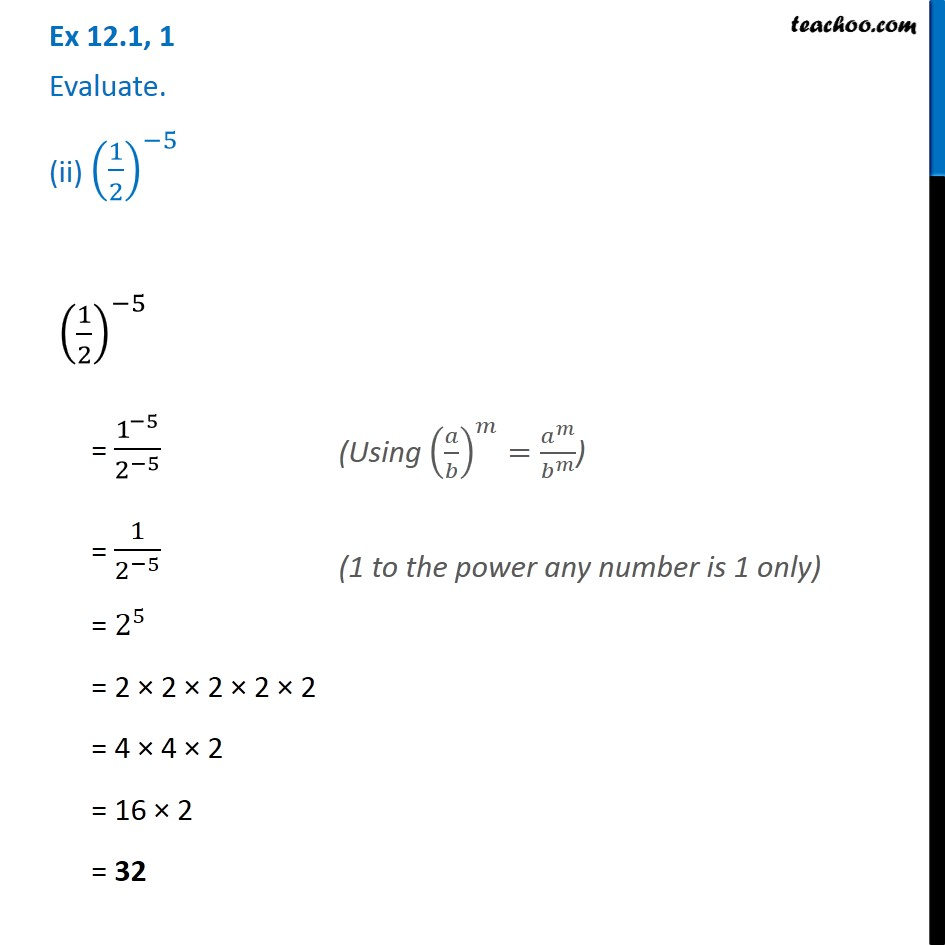Ex 12.1

Chapter 12 Class 8 Exponents and Powers
Serial order wiseIntroducing your new favourite teacher - Teachoo Black, at only ₹83 per month

### Transcript

Ex 12.1, 1 Evaluate. (ii) (1/2)^(−5) (1/2)^(−5) = 1^(−5)/2^(−5) = 1/2^(−5) = 2^5 = 2 × 2 × 2 × 2 × 2 = 4 × 4 × 2 = 16 × 2 = 32 (Using (𝑎/𝑏)^𝑚=𝑎^𝑚/𝑏^𝑚 ) (1 to the power any number is 1 only)